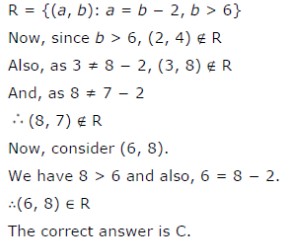# Let R be the relation in the set N given by R = {(a,b) : a = b - 2,b > 6} Choose the correct answer:

Let R be the relation in the set N given by R = {(a,b) : a = b - 2,b > 6} Choose the correct answer:
(A) (2, 4) R
(B) (3, 8) R
( C) (6, 8) R
(D) (8, 7) R